Processing ......FreeComputerBooks.com Links to Free Computer, Mathematics, Technical Books all over the World

Regression Models for Data Science in R
🌠 Top Free Unix/Linux Books - 100% Free or Open Source!
• Title: Regression Models for Data Science in R
• Author(s) Brian Caffo
• Publisher: Leanpub; eBook (Creative Commons Licensed)
• Hardcover/Paperback: N/A
• eBook: PDF
• Language: English
• ISBN-10/ASIN: N/A
• ISBN-13: N/A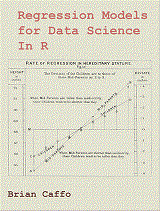Book Description

The ideal reader for this book will be quantitatively literate and has a basic understanding of statistical concepts and R programming. The student should have a basic understanding of statistical inference such as contained in https://leanpub.com/LittleInferenceBook/.

The book gives a rigorous treatment of the elementary concepts of Regression Models from a practical perspective. After reading the book and watching the associated videos, students will be able to perform multivariable regression models and understand their interpretations.

• N/A
Reviews, Rating, and Recommendations: Related Book Categories: Read and Download Links:Similar Books:
•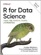R for Data Science: Visualize, Model, Transform, Tidy, Import

This book teaches you how to do data science with R: You'll learn how to get your data into R, get it into the most useful structure, transform it, visualize it and model it, how data science can help you work with the uncertainty and capture the opportunities.

•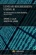Linear Regression Using R: An Introduction to Data Modeling

This book presents one of the fundamental data modeling techniques in an informal tutorial style. Learn how to predict system outputs from measured data using a detailed step-by-step process to develop, train, and test reliable regression models.

•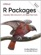R Packages: Organize, Test, Document, and Share Your Code

Turn your R code into packages that others can easily download and use. This practical book shows you how to bundle reusable R functions, sample data, and documentation together by applying author's package development philosophy.

•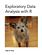Exploratory Data Analysis with R (Roger D. Peng)

This book covers the essential exploratory techniques for summarizing data with R. These techniques are typically applied before formal modeling commences and can help inform the development of more complex statistical models.

•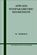Applied Nonparametric Regression (Wolfgang K. Hardle)

This book focuses on the applications and practical problems of two central aspects of curve smoothing: the choice of smoothing parameters and the construction of confidence bounds.

•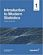Introduction to Modern Statistics (Mine Çetinkaya-Rundel, et al.)

This book puts a heavy emphasis on exploratory data analysis and provides a thorough discussion of simulation-based inference using randomization and bootstrapping, followed by a presentation of the related Central Limit Theorem based approaches.

•Foundations in Statistical Reasoning (Pete Kaslik)

This book is designed for students taking an introductory statistics class. The emphasis throughout the entire book is on how to make decisions with only partial evidence. It focuses on the thought process.

•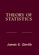Theory of Statistics (James E. Gentle)

This book is directed toward students for whom mathematical statistics is or will become an important part of their lives. It covers classical likelihood, Bayesian, and permutation inference; an introduction to basic asymptotic distribution theory; and modern topics.

•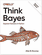O'Reilly® Think Bayes: Bayesian Statistics in Python

If you know how to program, you're ready to tackle Bayesian statistics. With this book, you'll learn how to solve statistical problems with Python code instead of mathematical formulas, using discrete probability distributions rather than continuous mathematics.

•Statistical Inference: Algorithms, Evidence, and Data Science

A masterful guide to how the inferential bases of classical statistics can provide a principled disciplinary frame for the data science of the twenty-first century. Every aspiring data scientist should carefully study this book, use it as a reference.

•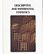Foundations of Descriptive and Inferential Statistics (H. van Elst)

This book aim to provide an accessible though technically solid introduction to the logic of systematical analyses of statistical data to both undergraduate and postgraduate students, in particular in the Social Sciences, Economics, and the Financial Services.

•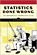Statistics Done Wrong: The Woefully Complete Guide (Reinhart)

Scientific progress depends on good research, and good research needs good statistics. But statistical analysis is tricky to get right, even for the best and brightest of us. You'd be surprised how many scientists are doing it wrong.

•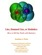Lies, Damned Lies: How to Tell the Truth with Statistics

The goal is to help you learn How to Tell the Truth with Statistics and, therefore, how to tell when others are telling the truth ... or are faking their "news". Covers Data Analysis, Binomial and normal models, Sample statistics, confidence intervals, hypothesis tests, etc.

•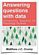Answering Questions with Data : Introductory Statistics

This is a free textbook teaching introductory statistics for undergraduates. Students will learn to select an appropriate data analysis technique, carry out the analysis, and draw appropriate conclusions.

Book Categories
 :All CategoriesTop Free BooksRecent BooksMiscellaneous BooksComputer EngineeringComputer LanguagesComputer ScienceData Science/DatabasesJava and Java EE (J2EE)Linux and UnixMathematicsMicrosoft and .NETMobile ComputingNetworking and CommunicationsSoftware EngineeringSpecial TopicsWeb Programming
Other Categories